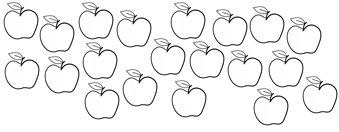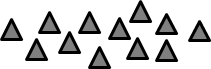Match Fishtank is now Fishtank Learning!

# Multiplication and Division, Part 1

## Objective

Identify and create situations involving an unknown number of groups and find the number of groups in situations.

## Common Core Standards

### Core Standards

?

• 3.OA.A.1 — Interpret products of whole numbers, e.g., interpret 5 × 7 as the total number of objects in 5 groups of 7 objects each. For example, describe a context in which a total number of objects can be expressed as 5 × 7.

• 3.OA.A.2 — Interpret whole-number quotients of whole numbers, e.g., interpret 56 ÷ 8 as the number of objects in each share when 56 objects are partitioned equally into 8 shares, or as a number of shares when 56 objects are partitioned into equal shares of 8 objects each. For example, describe a context in which a number of shares or a number of groups can be expressed as 56 ÷ 8.

• 3.OA.A.3 — Use multiplication and division within 100 to solve word problems in situations involving equal groups, arrays, and measurement quantities, e.g., by using drawings and equations with a symbol for the unknown number to represent the problem.

## Criteria for Success

?

1. Represent the number of groups and size of groups in a picture.
2. Divide a number of objects into groups of a given size in order to find the number of groups.
3. Write a multiplication equation to represent a given situation or picture where the unknown is the number of groups, including the number of rows of an array (MP.2).
4. Draw a picture to represent a given multiplication equation where the unknown is the number of groups, including the number of rows of an array (MP.2).

## Tips for Teachers

?

• The following material is needed for today's lesson: Counters

#### Remote Learning Guidance

If you need to adapt or shorten this lesson for remote learning, we suggest prioritizing Anchor Task 2 (benefits from worked example) and Anchor Task 3 (can be done independently). Find more guidance on adapting our math curriculum for remote learning here.

#### Fishtank Plus

• Problem Set
• Student Handout Editor
• Vocabulary Package

?

### Problem 1

Split 18 counters equally into groups of two.

a. How many counters do you have in each group?

b. Write a multiplication equation to represent this situation.

How is this similar to Anchor Task #1 from Lesson 4? How is it different?

### Problem 2

a. Ms. Holcombe picked the apples below this weekend. She wants to put 2 apples in a bag to give to each of her friends.i. Write a multiplication equation to represent this problem.

ii. How many bags of apples did Ms. Holcombe fill?

b. A grocery store puts 15 watermelons on display. They arrange them in rows of 5.

i. Write a multiplication equation to represent this problem.

ii. How many rows of watermelon are there?

### Problem 3

Draw a picture or write a story to represent this multiplication situation. Then find the solution.

___ $\times 4 = 12$

#### References

EngageNY Mathematics Grade 3 Mathematics > Module 1 > Topic B > Lesson 5Concept Development

Grade 3 Mathematics > Module 1 > Topic B > Lesson 5 of the New York State Common Core Mathematics Curriculum from EngageNY and Great Minds. © 2015 Great Minds. Licensed by EngageNY of the New York State Education Department under the CC BY-NC-SA 3.0 US license. Accessed Dec. 2, 2016, 5:15 p.m..

Modified by Fishtank Learning, Inc.

## Problem Set & Homework

#### Discussion of Problem Set

• What was similar about #8 and #9? What was different?
• How did your visual models in #11 and #12 help you to determine the unknown factor? Did anyone use a different strategy to solve?
• Did you “just know” the missing factor for any of the problems? Which one(s)?
• How is your approach to drawing a model to represent a problem with an unknown number of groups different from your approach to drawing a model to represent a problem with an unknown group size?
• How are the multiplication sentences we wrote today different from those we wrote yesterday? What explains this difference?

?

### Problem 1

Split 12 triangles into groups of 6 to find the number of groups.

____ $\times 6 = 12$#### References

EngageNY Mathematics Grade 3 Mathematics > Module 1 > Topic B > Lesson 5Exit Ticket, Question #1

Grade 3 Mathematics > Module 1 > Topic B > Lesson 5 of the New York State Common Core Mathematics Curriculum from EngageNY and Great Minds. © 2015 Great Minds. Licensed by EngageNY of the New York State Education Department under the CC BY-NC-SA 3.0 US license. Accessed Dec. 2, 2016, 5:15 p.m..

Modified by Fishtank Learning, Inc.

### Problem 2

Spencer buys 20 strawberries to make smoothies. Each smoothie needs 5 strawberries. How many smoothies can Spencer make? Show or explain your work.

#### References

EngageNY Mathematics Grade 3 Mathematics > Module 1 > Topic B > Lesson 5Exit Ticket, Question #2

Grade 3 Mathematics > Module 1 > Topic B > Lesson 5 of the New York State Common Core Mathematics Curriculum from EngageNY and Great Minds. © 2015 Great Minds. Licensed by EngageNY of the New York State Education Department under the CC BY-NC-SA 3.0 US license. Accessed Dec. 2, 2016, 5:15 p.m..

Modified by Fishtank Learning, Inc.

### Mastery Response

?